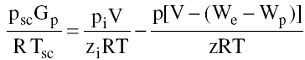# The material Balance Method Assignment Help

## The material Balance Method

If enough production-pressure history is available for a gas reservoir, the initial gas in place G, the initial reservoir pressure pi, and the gas reserves can be calculated without knowing A, h, f, or Sw. This is accomplished by forming a mass or mole balance on the gas as:

np = ni - nfWhere

np = moles of gas produced

ni = moles of gas initially in the reservoir

nf = moles of gas remaining in the reservoir.

## The material Balance Method Assignment Help By Online Tutoring and Guided Sessions from AssignmentHelp.Net

Using gas equation in above equation:Where

Pi = initial reservoir pressure

Gp = cumulative gas production, scf

P = current reservoir pressure

V = original gas volume, ft3

zi = gas deviation factor at pi

z = gas deviation factor at p

T = temperature, °R

We = cumulative water influx, ft3

Wp = cumulative water production, ft3